Intermediate Algebra 2e

# Key Concepts

### Key Concepts

#### 3.1Graph Linear Equations in Two Variables

• Points on the Axes
• Points with a y-coordinate equal to 0 are on the x-axis, and have coordinates $(a,0).(a,0).$
• Points with an x-coordinate equal to $00$ are on the y-axis, and have coordinates $(0,b).(0,b).$
$Quadrant IQuadrant IIQuadrant IIIQuadrant IV (x,y)(x,y)(x,y)(x,y) (+,+)(−,+)(−,−)(+,−) Quadrant IQuadrant IIQuadrant IIIQuadrant IV (x,y)(x,y)(x,y)(x,y) (+,+)(−,+)(−,−)(+,−)$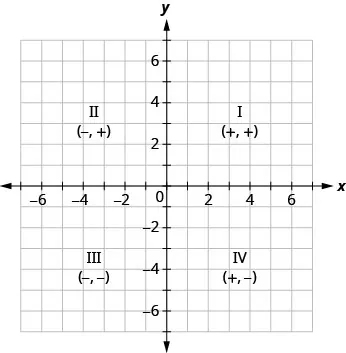• Graph of a Linear Equation: The graph of a linear equation $Ax+By=CAx+By=C$ is a straight line.
Every point on the line is a solution of the equation.
Every solution of this equation is a point on this line.
• How to graph a linear equation by plotting points.
1. Step 1. Find three points whose coordinates are solutions to the equation. Organize them in a table.
2. Step 2. Plot the points in a rectangular coordinate system. Check that the points line up. If they do not, carefully check your work.
3. Step 3. Draw the line through the three points. Extend the line to fill the grid and put arrows on both ends of the line.
• x-intercept and y-intercept of a Line
• The x-intercept is the point $(a,0)(a,0)$ where the line crosses the x-axis.
• The y-intercept is the point $(0,b)(0,b)$ where the line crosses the y-axis.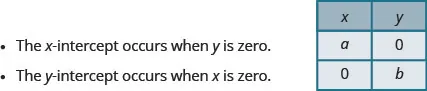• Find the x- and y-intercepts from the Equation of a Line
• Use the equation of the line. To find:
the x-intercept of the line, let $y=0y=0$ and solve for x.
the y-intercept of the line, let $x=0x=0$ and solve for y.
• How to graph a linear equation using the intercepts.
1. Step 1. Find the x- and y-intercepts of the line.
Let $y=0y=0$ and solve for x.
Let $x=0x=0$ and solve for y.
2. Step 2. Find a third solution to the equation.
3. Step 3. Plot the three points and check that they line up.
4. Step 4. Draw the line

#### 3.2Slope of a Line

• Slope of a Line
• The slope of a line is $m=riserun.m=riserun.$
• The rise measures the vertical change and the run measures the horizontal change.

• How to find the slope of a line from its graph using $m=riserun.m=riserun.$
1. Step 1. Locate two points on the line whose coordinates are integers.
2. Step 2. Starting with one point, sketch a right triangle, going from the first point to the second point.
3. Step 3. Count the rise and the run on the legs of the triangle.
4. Step 4. Take the ratio of rise to run to find the slope: $m=riserun.m=riserun.$
• Slope of a line between two points.
• The slope of the line between two points $(x1,y1)(x1,y1)$ and $(x2,y2)(x2,y2)$ is:
$m=y2−y1x2−x1.m=y2−y1x2−x1.$
• How to graph a line given a point and the slope.
1. Step 1. Plot the given point.
2. Step 2. Use the slope formula $m=riserunm=riserun$ to identify the rise and the run.
3. Step 3. Starting at the given point, count out the rise and run to mark the second point.
4. Step 4. Connect the points with a line.
• Slope Intercept Form of an Equation of a Line
• The slope–intercept form of an equation of a line with slope m and y-intercept, $(0,b)(0,b)$ is $y=mx+by=mx+b$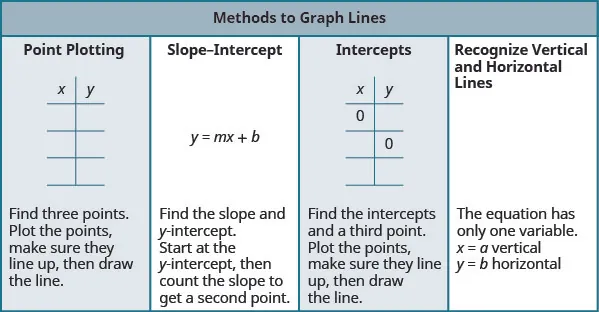• Parallel Lines
• Parallel lines are lines in the same plane that do not intersect.
Parallel lines have the same slope and different y-intercepts.
If $m1m1$ and $m2m2$ are the slopes of two parallel lines then $m1=m2.m1=m2.$
Parallel vertical lines have different x-intercepts.
• Perpendicular Lines
• Perpendicular lines are lines in the same plane that form a right angle.
• If $m1m1$ and $m2m2$ are the slopes of two perpendicular lines, then:
their slopes are negative reciprocals of each other, $m1=−1m2.m1=−1m2.$
the product of their slopes is $−1,−1,$$m1·m2=−1.m1·m2=−1.$
• A vertical line and a horizontal line are always perpendicular to each other.

#### 3.3Find the Equation of a Line

• How to find an equation of a line given the slope and a point.
1. Step 1. Identify the slope.
2. Step 2. Identify the point.
3. Step 3. Substitute the values into the point-slope form, $y−y1=m(x−x1).y−y1=m(x−x1).$
4. Step 4. Write the equation in slope-intercept form.

• How to find an equation of a line given two points.
1. Step 1. Find the slope using the given points. $m=y2−y1x2−x1m=y2−y1x2−x1$
2. Step 2. Choose one point.
3. Step 3. Substitute the values into the point-slope form: $y−y1=m(x−x1).y−y1=m(x−x1).$
4. Step 4.
Write the equation in slope-intercept form.
To Write an Equation of a Line
If given: Use: Form:
Slope and y-intercept slope-intercept $y=mx+by=mx+b$
Slope and a point point-slope $y−y1=m(x−x1)y−y1=m(x−x1)$
Two points point-slope $y−y1=m(x−x1)y−y1=m(x−x1)$
• How to find an equation of a line parallel to a given line.
1. Step 1. Find the slope of the given line.
2. Step 2. Find the slope of the parallel line.
3. Step 3. Identify the point.
4. Step 4. Substitute the values into the point-slope form: $y−y1=m(x−x1).y−y1=m(x−x1).$
5. Step 5. Write the equation in slope-intercept form
• How to find an equation of a line perpendicular to a given line.
1. Step 1. Find the slope of the given line.
2. Step 2. Find the slope of the perpendicular line.
3. Step 3. Identify the point.
4. Step 4. Substitute the values into the point-slope form, $y−y1=m(x−x1)y−y1=m(x−x1)$
5. Step 5. Write the equation in slope-intercept form.

#### 3.4Graph Linear Inequalities in Two Variables

• How to graph a linear inequality in two variables.
1. Step 1. Identify and graph the boundary line.
If the inequality is $≤or≥,≤or≥,$ the boundary line is solid.
If the inequality is $,,$ the boundary line is dashed.
2. Step 2. Test a point that is not on the boundary line. Is it a solution of the inequality?
3. Step 3. Shade in one side of the boundary line.
If the test point is a solution, shade in the side that includes the point.
If the test point is not a solution, shade in the opposite side.

#### 3.5Relations and Functions

• Function Notation: For the function $y=f(x)y=f(x)$
• f is the name of the function
• x is the domain value
• $f(x)f(x)$ is the range value y corresponding to the value x
We read $f(x)f(x)$ as f of x or the value of f at x.
• Independent and Dependent Variables: For the function $y=f(x),y=f(x),$
• x is the independent variable as it can be any value in the domain
• y is the dependent variable as its value depends on x

#### 3.6Graphs of Functions

• Vertical Line Test
• A set of points in a rectangular coordinate system is the graph of a function if every vertical line intersects the graph in at most one point.
• If any vertical line intersects the graph in more than one point, the graph does not represent a function.
• Graph of a Function
• The graph of a function is the graph of all its ordered pairs, $(x,y)(x,y)$ or using function notation, $(x,f(x))(x,f(x))$ where $y=f(x).y=f(x).$
$fname of functionxx-coordinate of the ordered pairf(x)y-coordinate of the ordered pairfname of functionxx-coordinate of the ordered pairf(x)y-coordinate of the ordered pair$
• Linear Function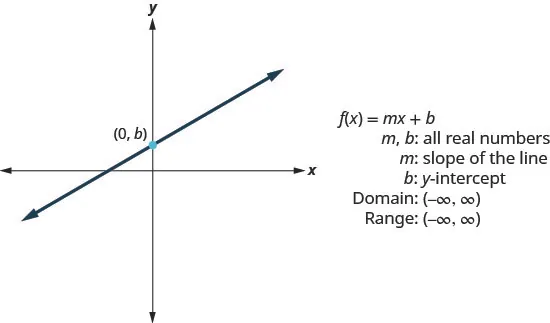• Constant Function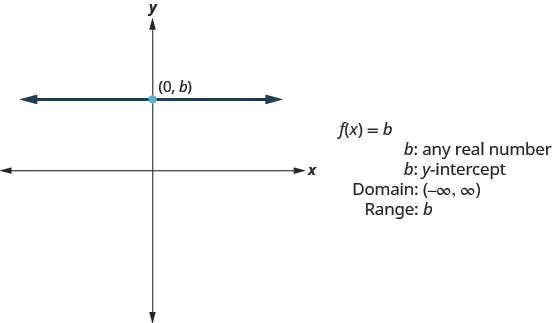• Identity Function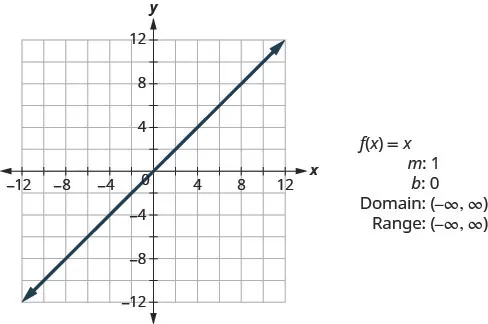• Square Function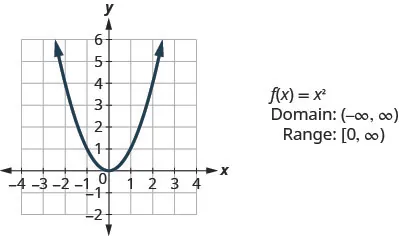• Cube Function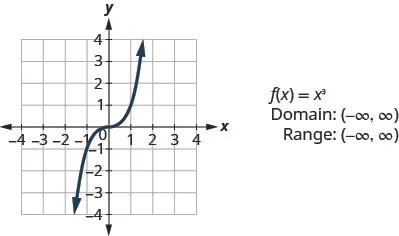• Square Root Function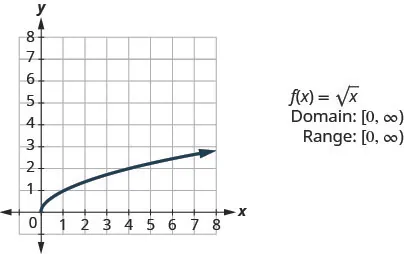• Absolute Value Function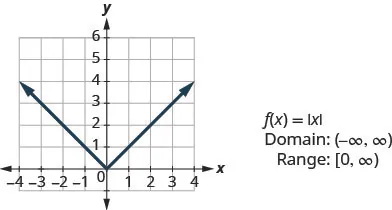Order a print copy

As an Amazon Associate we earn from qualifying purchases.